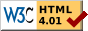## Counting in base 20 using underlined digits

#### by Guillaume Lathoud, June 2012

Digits 0 to 9 have the same meaning as in base 10. Underlined digits 0 to 9 correspond to numbers 10 to 19 in base 10. If you stare long enough at the multiplication table, you may find interesting patterns.

Things get interesting when you try to mentally compute multiplications. Here are some crutches.

### Update 2015-02-25

If you like this, you may also like balanced base 20, where the underline has a different meaning, more like a negative sign. Here's why and how.

``docWriteOperationTable('+')``

### Substraction

``docWriteOperationTable('-')``

### Multiplication

``docWriteOperationTable('*')``

### Some mental crutches

``````a * b

When a > _0 and b > _0:

_3 * _4 = (_0 + 3) * (_0 + 4)
= _0 * (_0 + 3 + 4) + 3 * 4
= _0 * _7           + _2
= 8_0              + _2
= 92

in particular, when a+b = 1_0  (30 in base 10)
_1 * _9 = _00 + 1 * 9 = _09
_2 * _8 = _00 + 2 * 8 = _0_6
_3 * _7 = _00 + 3 * 7 = _11
_4 * _6 = _00 + 4 * 6 = _14
_5 * _5 = _00 + 5 * 5 = _15

and when a = b
_1 * _1 = (5 + 1) * 10 + 1 * 1 = 61
_2 * _2 = (5 + 2) * 10 + 2 * 2 = 74
_3 * _3 = (5 + 3) * 10 + 3 * 3 = 89
_4 * _4 = (5 + 4) * 10 + 4 * 4 = 9_6
_5 * _5 = (5 + 5) * 10 + 5 * 5 = _15
_6 * _6 = (5 + 6) * 10 + 6 * 6 = _10 + 1_6 = _2_6  (256 in base 10)
_7 * _7 = (5 + 7) * 10 + 7 * 7 = _20 + 29 = _49
_8 * _8 = (5 + 8) * 10 + 8 * 8 = _30 + 34 = _64
_9 * _9 = (5 + 9) * 10 + 9 * 9 = _40 + 41 = _81

and when (a+b)/2 is an integer
_5 * _7 = (_6 - 1) * (_6 + 1) = _6 * _6 - 1 * 1 = _2_6 - 1 = _2_5  (255 in base 10)
_4 * _8 = _6^2 - 2^2 = _2_6 - 4 = _2_2
_3 * _9 = _6^2 - 3^2 = _2_6 - 9 = _17
``````

### You might also like

`log10circle`: circles on paper for quick computer-free calculations: multiplication, division, power, sine, cosine.

`cipo` investigates a property of circular sets of 2^q elements.`hashfiddle` lets you play with JavaScript, HTML and CSS fully online, without any server.

Produced on 2017-05-10 by index.scm - by Guillaume Lathoud (glat _at_ glat _dot_ info)